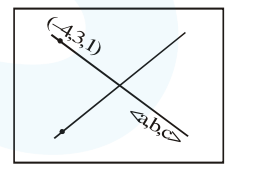# The equation of the line passing through

Question:

The equation of the line passing through $(-4,3,1)$, parallel to the plane $x+2 y-z-5=0$

and intersecting the line $\frac{x+1}{-3}=\frac{y-3}{2}=\frac{z-2}{-1}$ is:

1. $\frac{x+4}{-1}=\frac{y-3}{1}=\frac{z-1}{1}$

2. $\frac{x+4}{3}=\frac{y-3}{-1}=\frac{z-1}{1}$

3. $\frac{x+4}{1}=\frac{y-3}{1}=\frac{z-1}{3}$

4. $\frac{x-4}{2}=\frac{y+3}{1}=\frac{z+1}{4}$

Correct Option: , 2

Solution:Normal vector of plane containing two intersecting lines is parallel to vector.

$\left(\overrightarrow{\mathrm{V}}_{1}\right)=\left|\begin{array}{ccc}\hat{\mathrm{i}} & \hat{\mathrm{j}} & \hat{\mathrm{k}} \\ 3 & 0 & 1 \\ -3 & 2 & -1\end{array}\right|$

$=-2 \hat{\mathrm{i}}+6 \hat{\mathrm{k}}$

$\therefore$ Required line is parallel to vector

$\left(\overrightarrow{\mathrm{V}}_{2}\right)=\left|\begin{array}{ccc}\hat{\mathrm{i}} & \hat{\mathrm{j}} & \hat{\mathrm{k}} \\ 1 & 2 & -1 \\ -2 & 0 & 6\end{array}\right|=3 \hat{\mathrm{i}}-\hat{\mathrm{j}}+\hat{\mathrm{k}}$

$\Rightarrow$ Required equation of line is

$\frac{x+4}{3}=\frac{y-3}{-1}=\frac{z-1}{1}$